# Selina Solutions Concise Maths Class 7 Chapter 5: Exponents (Including Laws of Exponents) Exercise 5A

Selina Solutions Concise Maths Class 7 Chapter 5 Exponents (Including Laws of Exponents) Exercise 5A has problems on index or exponent and exponential form of a number. Numerous solved examples are present before each exercise to help students analyse the type of problems that would appear in the annual exam. Scoring good marks in a subject like Mathematics requires regular practise. Students gain a hold on the concepts, which are covered in the chapter, based on the latest syllabus. Selina Solutions Concise Maths Class 7 Chapter 5 Exponents (Including Laws of Exponents) Exercise 5A PDF links are given here with a free download option.

## Selina Solutions Concise Maths Class 7 Chapter 5: Exponents (Including Laws of Exponents) Exercise 5A Download PDF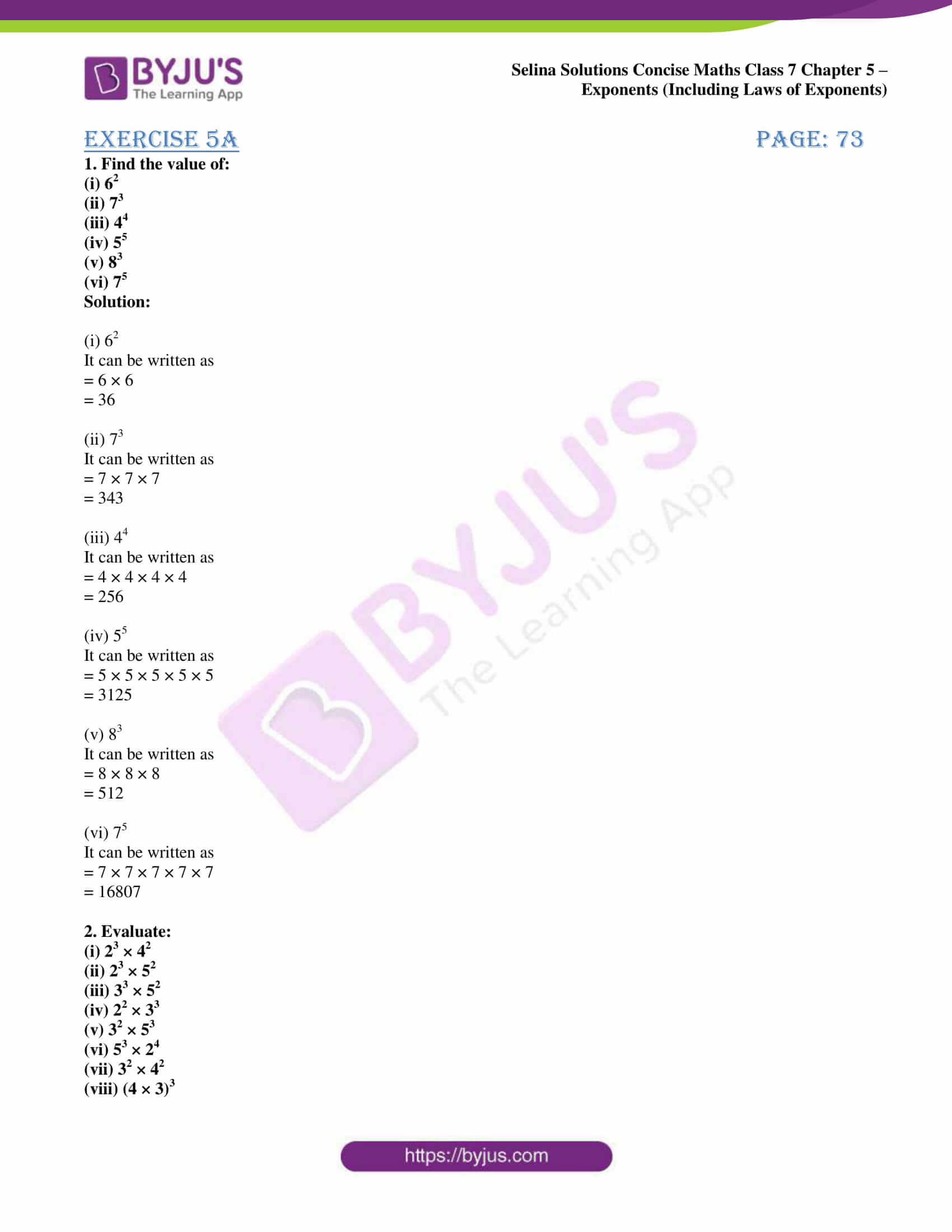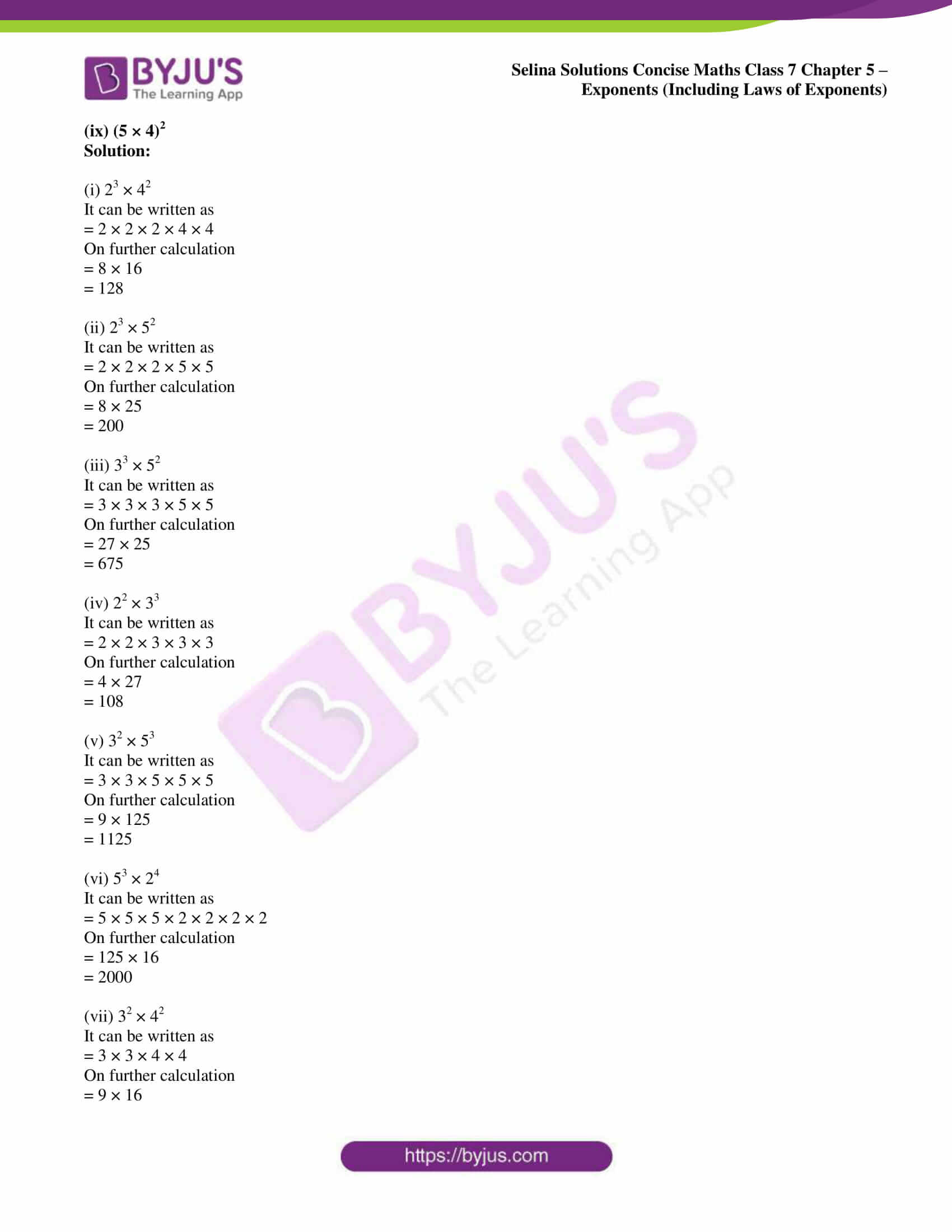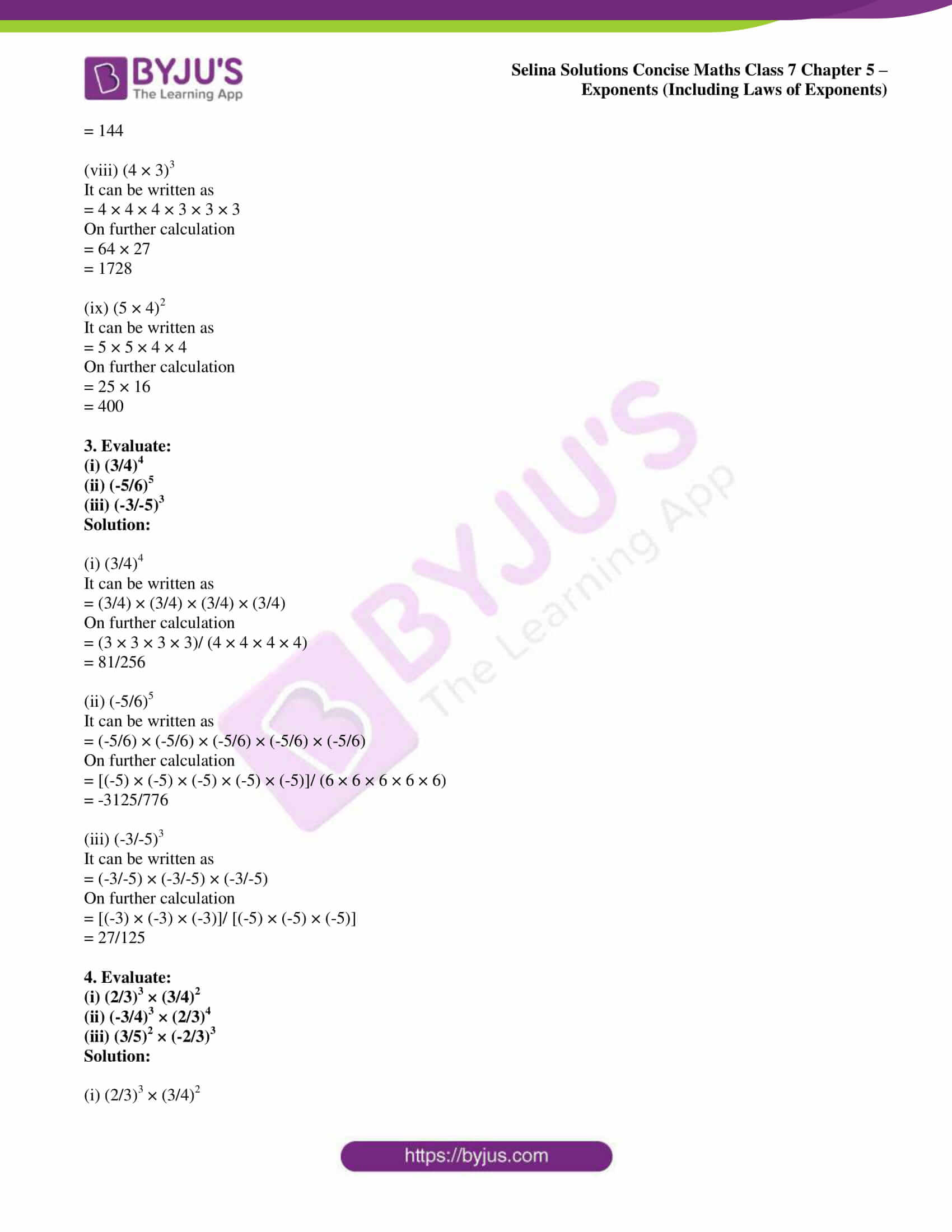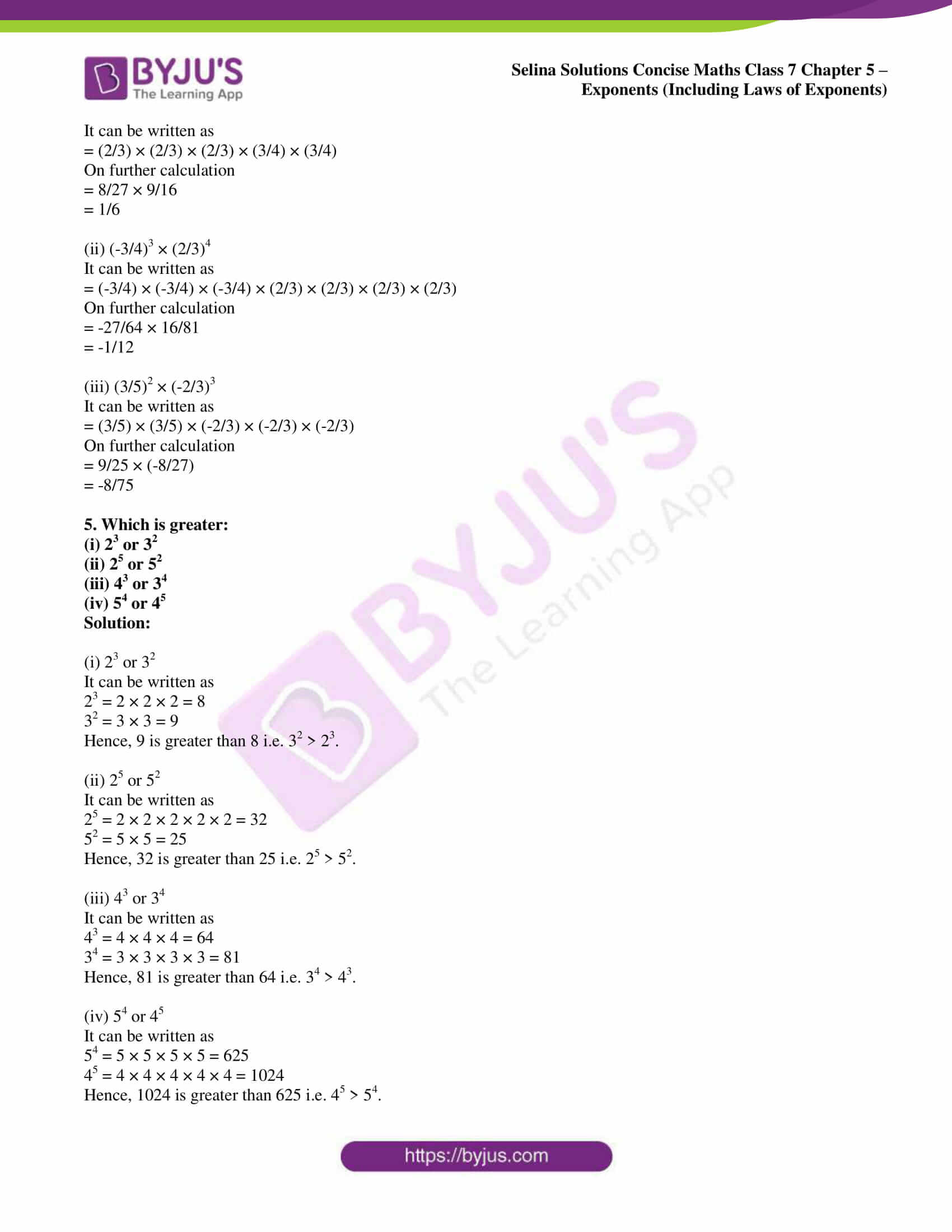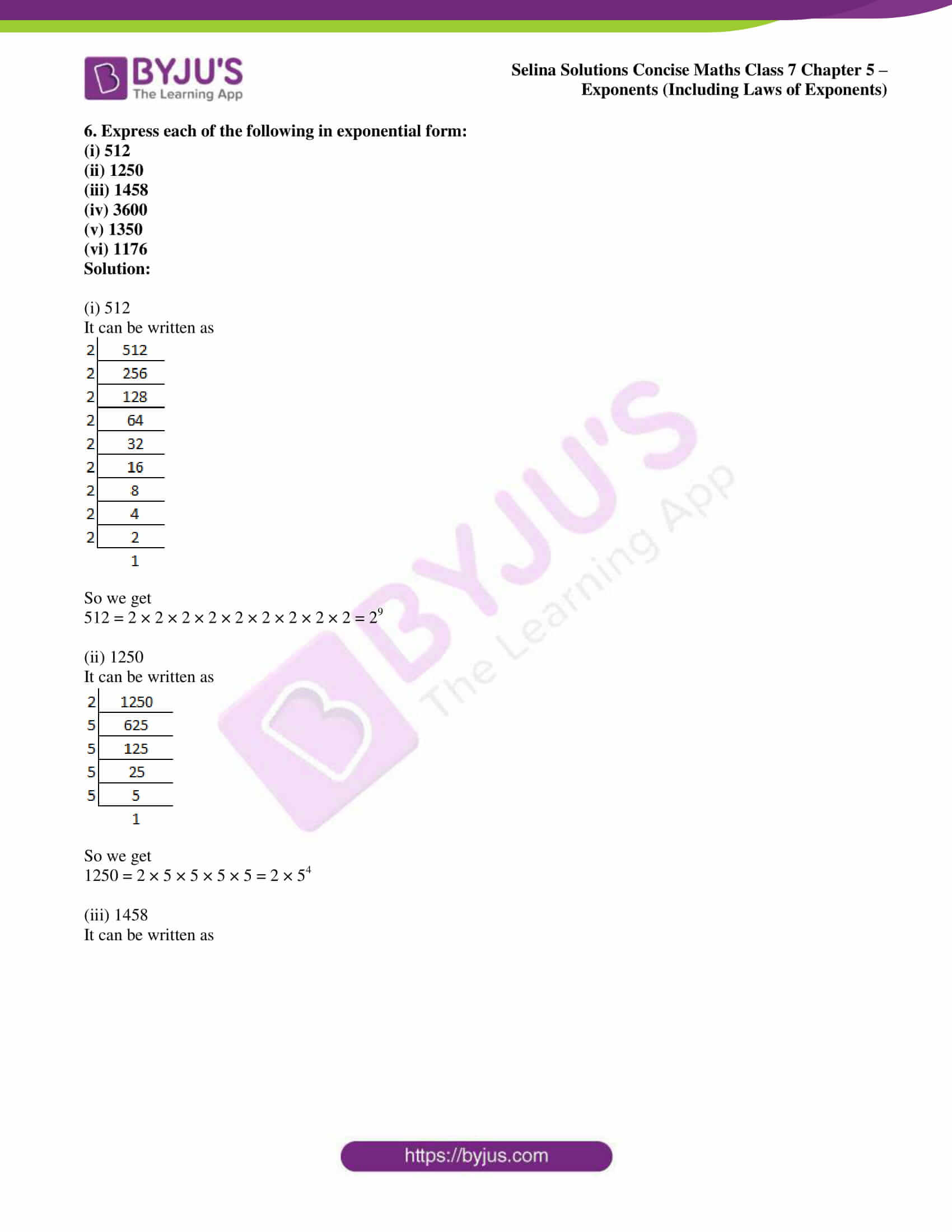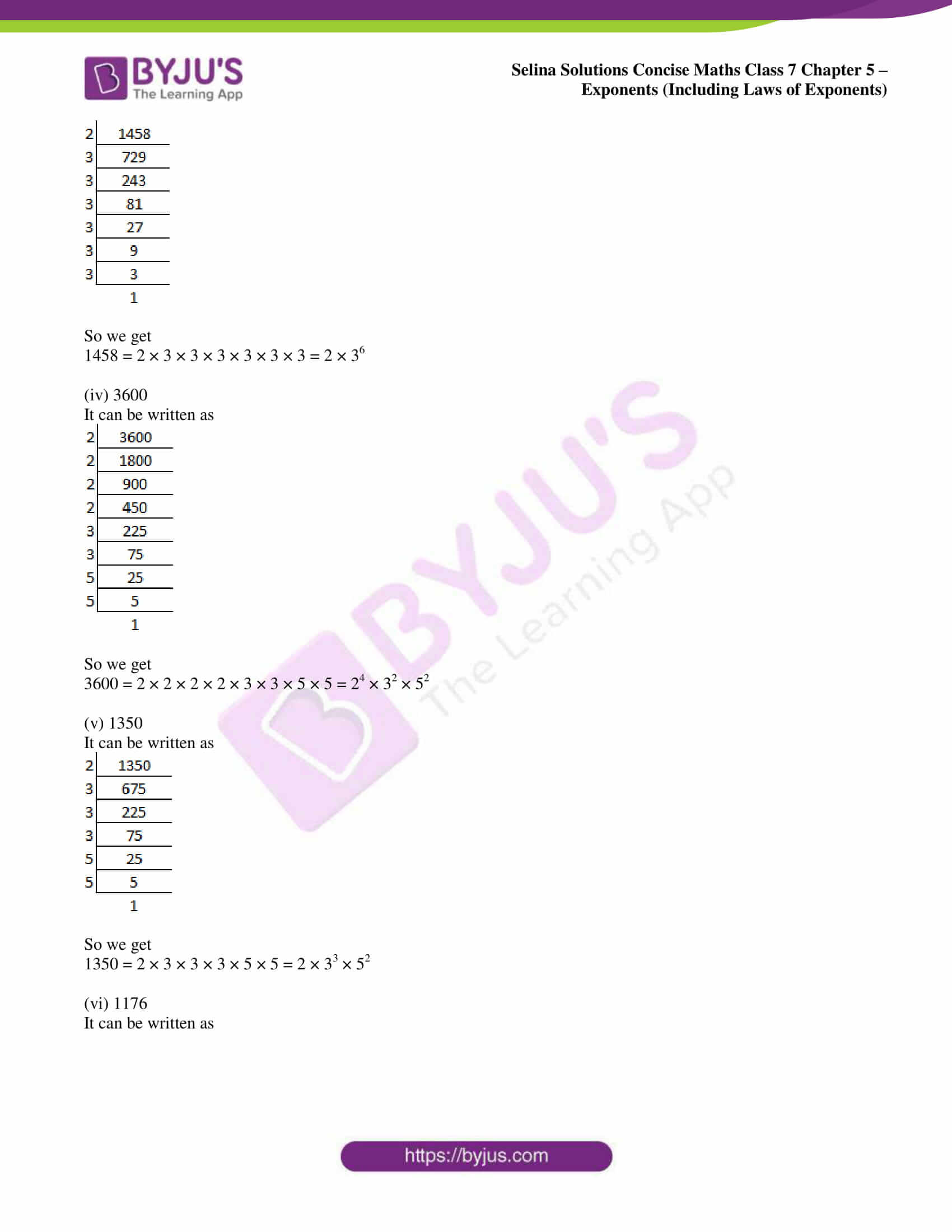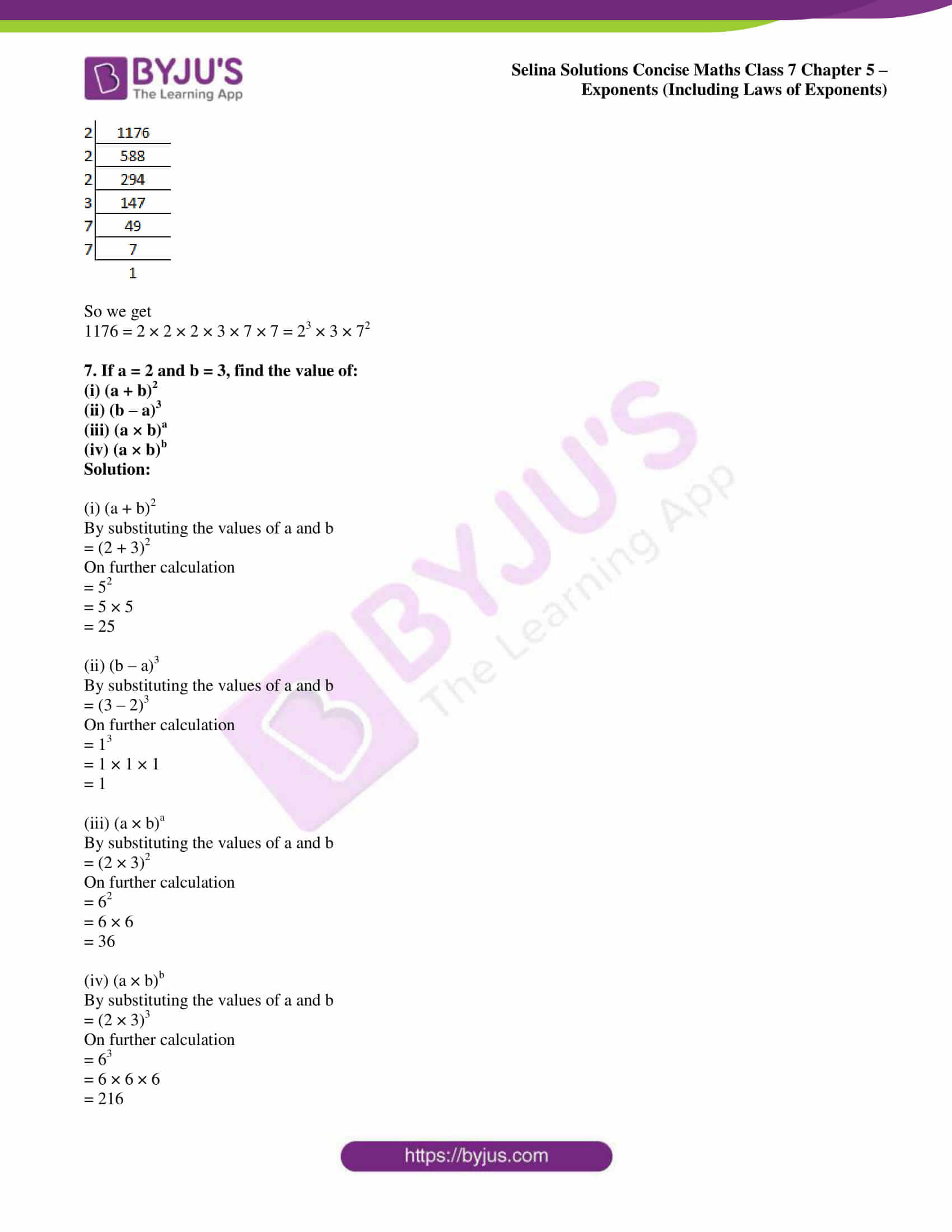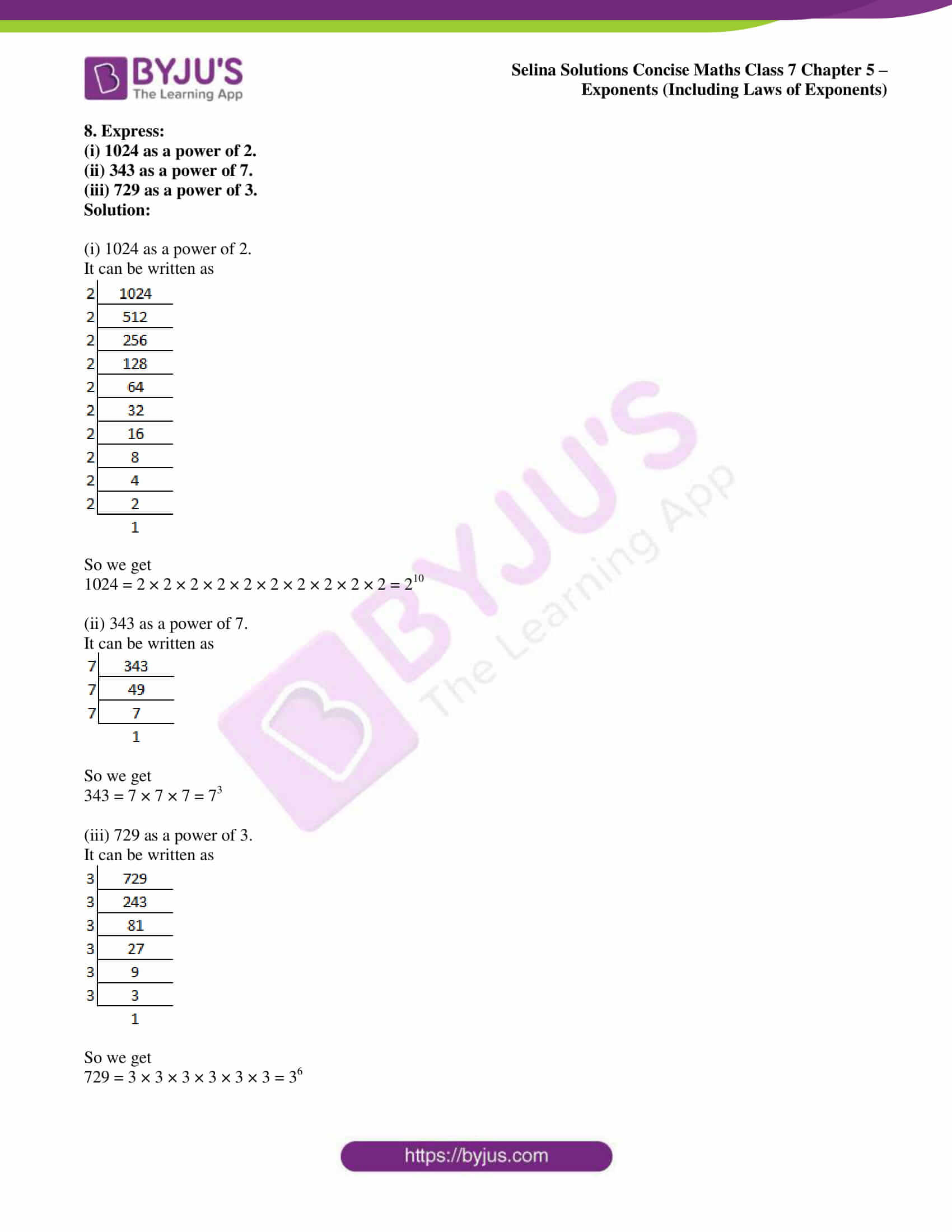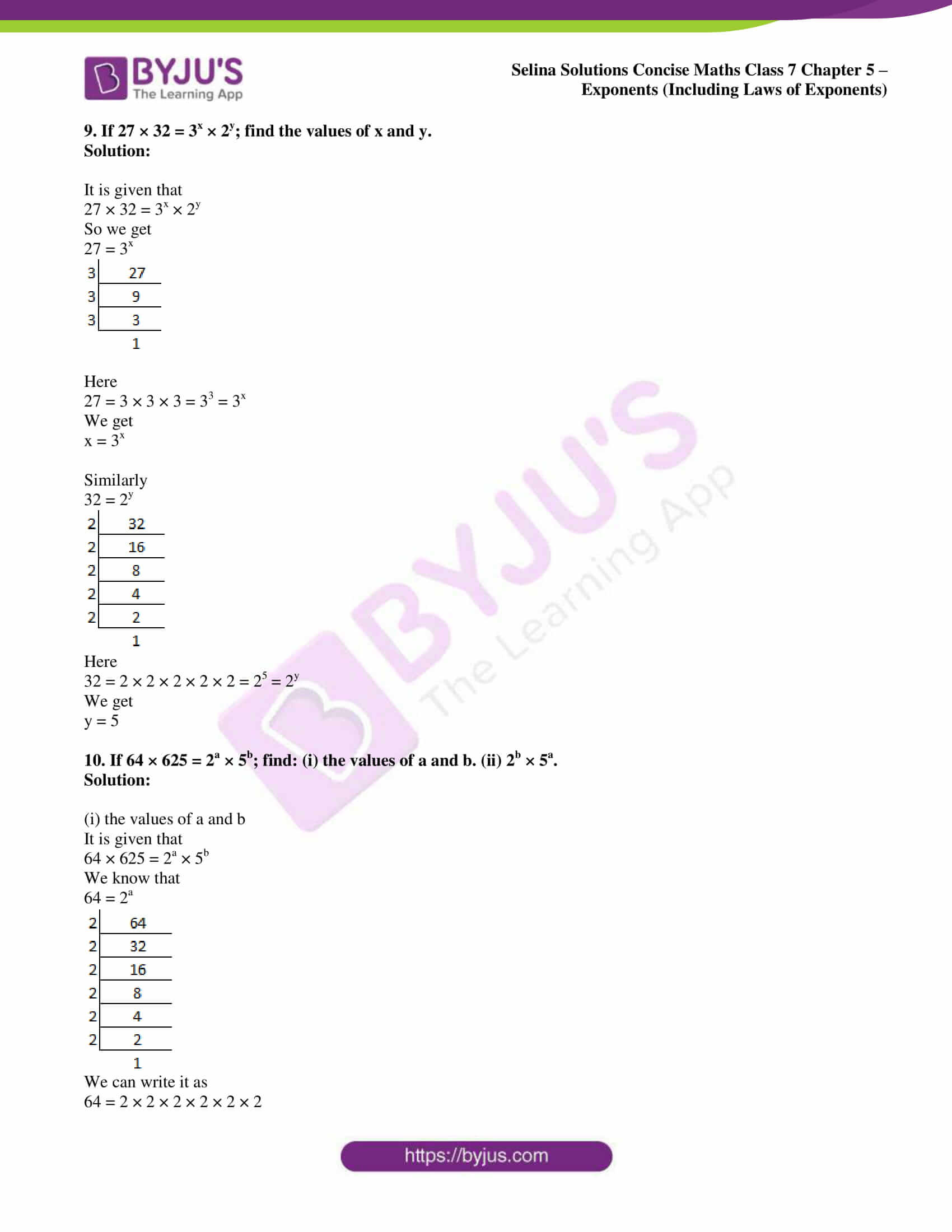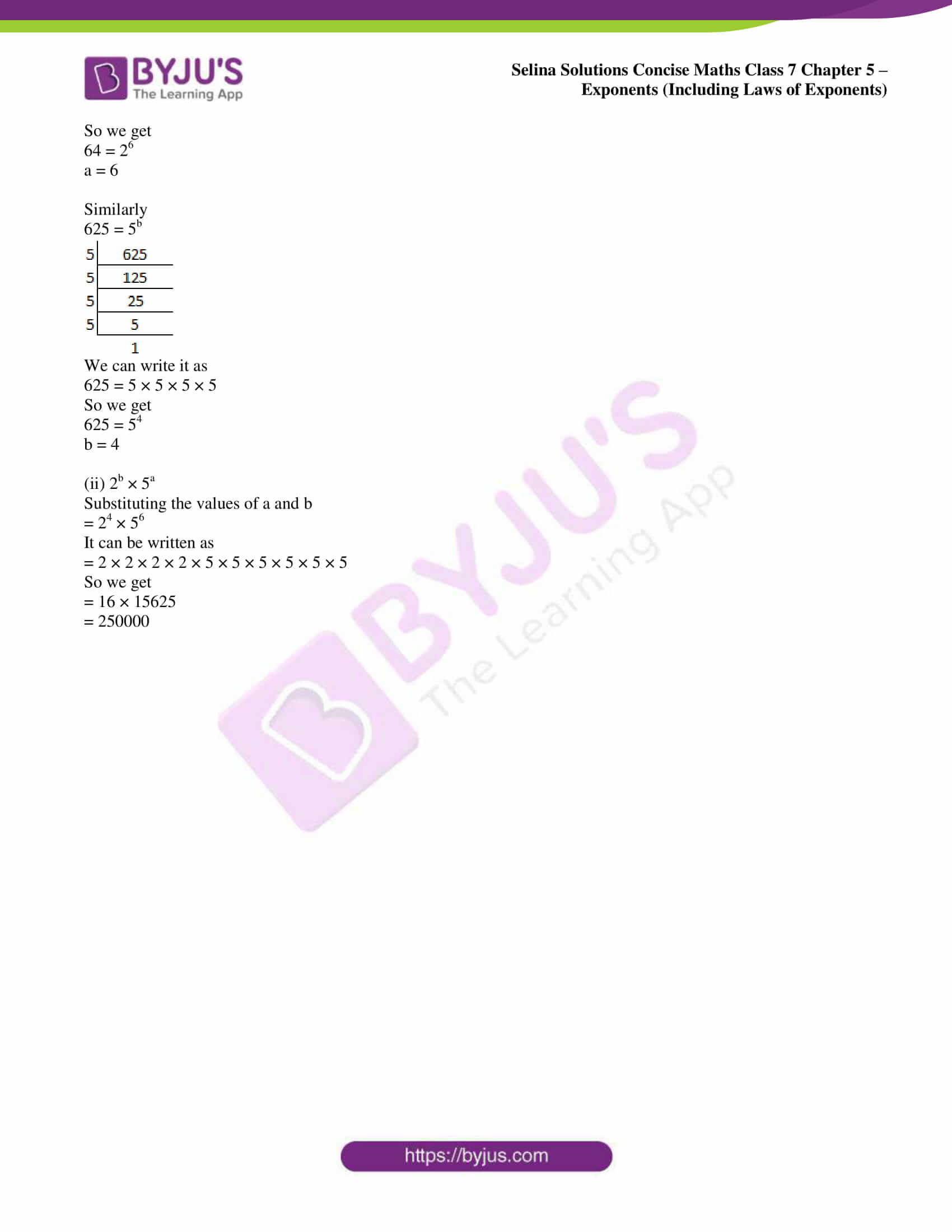### Access Selina Solutions Concise Maths Class 7 Chapter 5: Exponents (Including Laws of Exponents) Exercise 5A

1. Find the value of:

(i) 62

(ii) 73

(iii) 44

(iv) 55

(v) 83

(vi) 75

Solution:

(i) 62

It can be written as

= 6 × 6

= 36

(ii) 73

It can be written as

= 7 × 7 × 7

= 343

(iii) 44

It can be written as

= 4 × 4 × 4 × 4

= 256

(iv) 55

It can be written as

= 5 × 5 × 5 × 5 × 5

= 3125

(v) 83

It can be written as

= 8 × 8 × 8

= 512

(vi) 75

It can be written as

= 7 × 7 × 7 × 7 × 7

= 16807

2. Evaluate:

(i) 23 × 42

(ii) 23 × 52

(iii) 33 × 52

(iv) 22 × 33

(v) 32 × 53

(vi) 53 × 24

(vii) 32 × 42

(viii) (4 × 3)3

(ix) (5 × 4)2

Solution:

(i) 23 × 42

It can be written as

= 2 × 2 × 2 × 4 × 4

On further calculation

= 8 × 16

= 128

(ii) 23 × 52

It can be written as

= 2 × 2 × 2 × 5 × 5

On further calculation

= 8 × 25

= 200

(iii) 33 × 52

It can be written as

= 3 × 3 × 3 × 5 × 5

On further calculation

= 27 × 25

= 675

(iv) 22 × 33

It can be written as

= 2 × 2 × 3 × 3 × 3

On further calculation

= 4 × 27

= 108

(v) 32 × 53

It can be written as

= 3 × 3 × 5 × 5 × 5

On further calculation

= 9 × 125

= 1125

(vi) 53 × 24

It can be written as

= 5 × 5 × 5 × 2 × 2 × 2 × 2

On further calculation

= 125 × 16

= 2000

(vii) 32 × 42

It can be written as

= 3 × 3 × 4 × 4

On further calculation

= 9 × 16

= 144

(viii) (4 × 3)3

It can be written as

= 4 × 4 × 4 × 3 × 3 × 3

On further calculation

= 64 × 27

= 1728

(ix) (5 × 4)2

It can be written as

= 5 × 5 × 4 × 4

On further calculation

= 25 × 16

= 400

3. Evaluate:

(i) (3/4)4

(ii) (-5/6)5

(iii) (-3/-5)3

Solution:

(i) (3/4)4

It can be written as

= (3/4) × (3/4) × (3/4) × (3/4)

On further calculation

= (3 × 3 × 3 × 3)/ (4 × 4 × 4 × 4)

= 81/256

(ii) (-5/6)5

It can be written as

= (-5/6) × (-5/6) × (-5/6) × (-5/6) × (-5/6)

On further calculation

= [(-5) × (-5) × (-5) × (-5) × (-5)]/ (6 × 6 × 6 × 6 × 6)

= -3125/776

(iii) (-3/-5)3

It can be written as

= (-3/-5) × (-3/-5) × (-3/-5)

On further calculation

= [(-3) × (-3) × (-3)]/ [(-5) × (-5) × (-5)]

= 27/125

4. Evaluate:

(i) (2/3)3 × (3/4)2

(ii) (-3/4)3 × (2/3)4

(iii) (3/5)2 × (-2/3)3

Solution:

(i) (2/3)3 × (3/4)2

It can be written as

= (2/3) × (2/3) × (2/3) × (3/4) × (3/4)

On further calculation

= 8/27 × 9/16

= 1/6

(ii) (-3/4)3 × (2/3)4

It can be written as

= (-3/4) × (-3/4) × (-3/4) × (2/3) × (2/3) × (2/3) × (2/3)

On further calculation

= -27/64 × 16/81

= -1/12

(iii) (3/5)2 × (-2/3)3

It can be written as

= (3/5) × (3/5) × (-2/3) × (-2/3) × (-2/3)

On further calculation

= 9/25 × (-8/27)

= -8/75

5. Which is greater:

(i) 23 or 32

(ii) 25 or 52

(iii) 43 or 34

(iv) 54 or 45

Solution:

(i) 23 or 32

It can be written as

23 = 2 × 2 × 2 = 8

32 = 3 × 3 = 9

Hence, 9 is greater than 8 i.e. 32 > 23.

(ii) 25 or 52

It can be written as

25 = 2 × 2 × 2 × 2 × 2 = 32

52 = 5 × 5 = 25

Hence, 32 is greater than 25 i.e. 25 > 52.

(iii) 43 or 34

It can be written as

43 = 4 × 4 × 4 = 64

34 = 3 × 3 × 3 × 3 = 81

Hence, 81 is greater than 64 i.e. 34 > 43.

(iv) 54 or 45

It can be written as

54 = 5 × 5 × 5 × 5 = 625

45 = 4 × 4 × 4 × 4 × 4 = 1024

Hence, 1024 is greater than 625 i.e. 45 > 54.

6. Express each of the following in exponential form:

(i) 512

(ii) 1250

(iii) 1458

(iv) 3600

(v) 1350

(vi) 1176

Solution:

(i) 512

It can be written as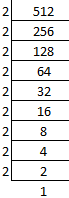So we get

512 = 2 × 2 × 2 × 2 × 2 × 2 × 2 × 2 × 2 = 29

(ii) 1250

It can be written as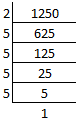So we get

1250 = 2 × 5 × 5 × 5 × 5 = 2 × 54

(iii) 1458

It can be written as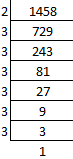So we get

1458 = 2 × 3 × 3 × 3 × 3 × 3 × 3 = 2 × 36

(iv) 3600

It can be written as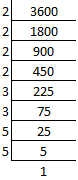So we get

3600 = 2 × 2 × 2 × 2 × 3 × 3 × 5 × 5 = 24 × 32 × 52

(v) 1350

It can be written as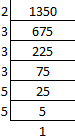So we get

1350 = 2 × 3 × 3 × 3 × 5 × 5 = 2 × 33 × 52

(vi) 1176

It can be written as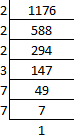So we get

1176 = 2 × 2 × 2 × 3 × 7 × 7 = 23 × 3 × 72

7. If a = 2 and b = 3, find the value of:

(i) (a + b)2

(ii) (b – a)3

(iii) (a × b)a

(iv) (a × b)b

Solution:

(i) (a + b)2

By substituting the values of a and b

= (2 + 3)2

On further calculation

= 52

= 5 × 5

= 25

(ii) (b – a)3

By substituting the values of a and b

= (3 – 2)3

On further calculation

= 13

= 1 × 1 × 1

= 1

(iii) (a × b)a

By substituting the values of a and b

= (2 × 3)2

On further calculation

= 62

= 6 × 6

= 36

(iv) (a × b)b

By substituting the values of a and b

= (2 × 3)3

On further calculation

= 63

= 6 × 6 × 6

= 216

8. Express:

(i) 1024 as a power of 2.

(ii) 343 as a power of 7.

(iii) 729 as a power of 3.

Solution:

(i) 1024 as a power of 2.

It can be written as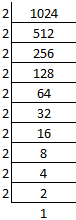So we get

1024 = 2 × 2 × 2 × 2 × 2 × 2 × 2 × 2 × 2 × 2 = 210

(ii) 343 as a power of 7.

It can be written as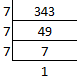So we get

343 = 7 × 7 × 7 = 73

(iii) 729 as a power of 3.

It can be written as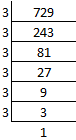So we get

729 = 3 × 3 × 3 × 3 × 3 × 3 = 36

9. If 27 × 32 = 3x × 2y; find the values of x and y.

Solution:

It is given that

27 × 32 = 3x × 2y

So we get

27 = 3x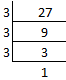Here

27 = 3 × 3 × 3 = 33 = 3x

We get

x = 3x

Similarly

32 = 2y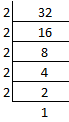Here

32 = 2 × 2 × 2 × 2 × 2 = 25 = 2y

We get

y = 5

10. If 64 × 625 = 2a × 5b; find: (i) the values of a and b. (ii) 2b × 5a.

Solution:

(i) the values of a and b

It is given that

64 × 625 = 2a × 5b

We know that

64 = 2a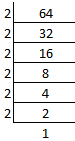We can write it as

64 = 2 × 2 × 2 × 2 × 2 × 2

So we get

64 = 26

a = 6

Similarly

625 = 5b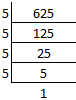We can write it as

625 = 5 × 5 × 5 × 5

So we get

625 = 54

b = 4

(ii) 2b × 5a

Substituting the values of a and b

= 24 × 56

It can be written as

= 2 × 2 × 2 × 2 × 5 × 5 × 5 × 5 × 5 × 5

So we get

= 16 × 15625

= 250000